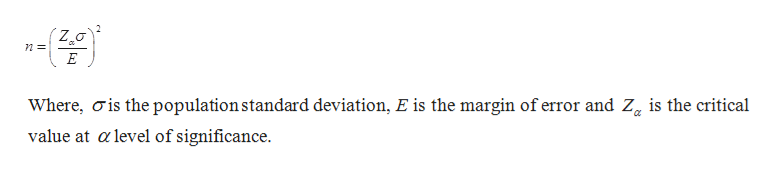# A research group wishes to estimate the mean number of hours that high school students spend watching TV on a weekday. A margin of error ofE=0.20 hour is desired. Past studies suggest that a population standard deviation ofσ=2.62.6 hours is reasonable. Estimate the minimum sample size required to estimate the population mean with​ 95% confidence. The required sample size is________.​(Round up to the nearest whole​ number.)

Question
2 views
A research group wishes to estimate the mean number of hours that high school students spend watching TV on a weekday. A margin of error of
E=0.20 hour is desired. Past studies suggest that a population standard deviation of
σ=2.62.6 hours is reasonable.

Estimate the minimum sample size required to estimate the population mean with​ 95% confidence.

The required sample size is
________.
​(Round up to the nearest whole​ number.)
check_circle

Step 1

The sample size formula is given below:help_outlineImage TranscriptioncloseWhere, ois the population standard deviation, E is the margin of error and Z, is the critical value at a level of significance. fullscreen
Step 2

It is given that the margin of error is 0.20 and the standard deviation is 2.62.

From the table of&...

### Want to see the full answer?

See Solution

#### Want to see this answer and more?

Solutions are written by subject experts who are available 24/7. Questions are typically answered within 1 hour.*

See Solution
*Response times may vary by subject and question.
Tagged in

### Other